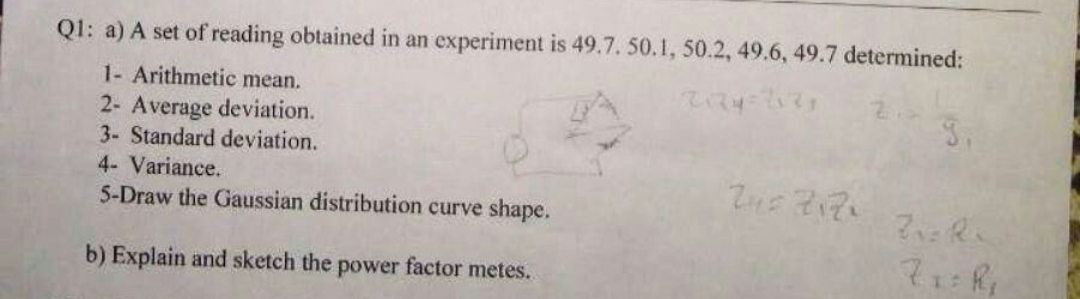# Ql: a) A set of reading obtained in an experiment is 49.7. 50.1, 50.2, 49.6, 49.7 determined:1- Arithmetic mean.2- Average deviation3- Standard deviation.4- Variance.5-Draw the Gaussian distribution curve shape.b) Explain and sketch the power factor metes.

Question
10 viewshelp_outlineImage TranscriptioncloseQl: a) A set of reading obtained in an experiment is 49.7. 50.1, 50.2, 49.6, 49.7 determined: 1- Arithmetic mean. 2- Average deviation 3- Standard deviation. 4- Variance. 5-Draw the Gaussian distribution curve shape. b) Explain and sketch the power factor metes. fullscreen
check_circle

Step 1

As this question has many sub parts, so I will be answering the first five sub-parts of Q1. a.   In case you need the answer of Q1. b, please post this as a new request.

(a)  1. Arithmetic Mean for the readings :49.7. 50.1, 50.2, 49.6, 49.7

Step 2

(2) Average Deviation:

Step 3

(3) Standard De...

### Want to see the full answer?

See Solution

#### Want to see this answer and more?

Solutions are written by subject experts who are available 24/7. Questions are typically answered within 1 hour.*

See Solution
*Response times may vary by subject and question.
Tagged in

### Electrical Engineering